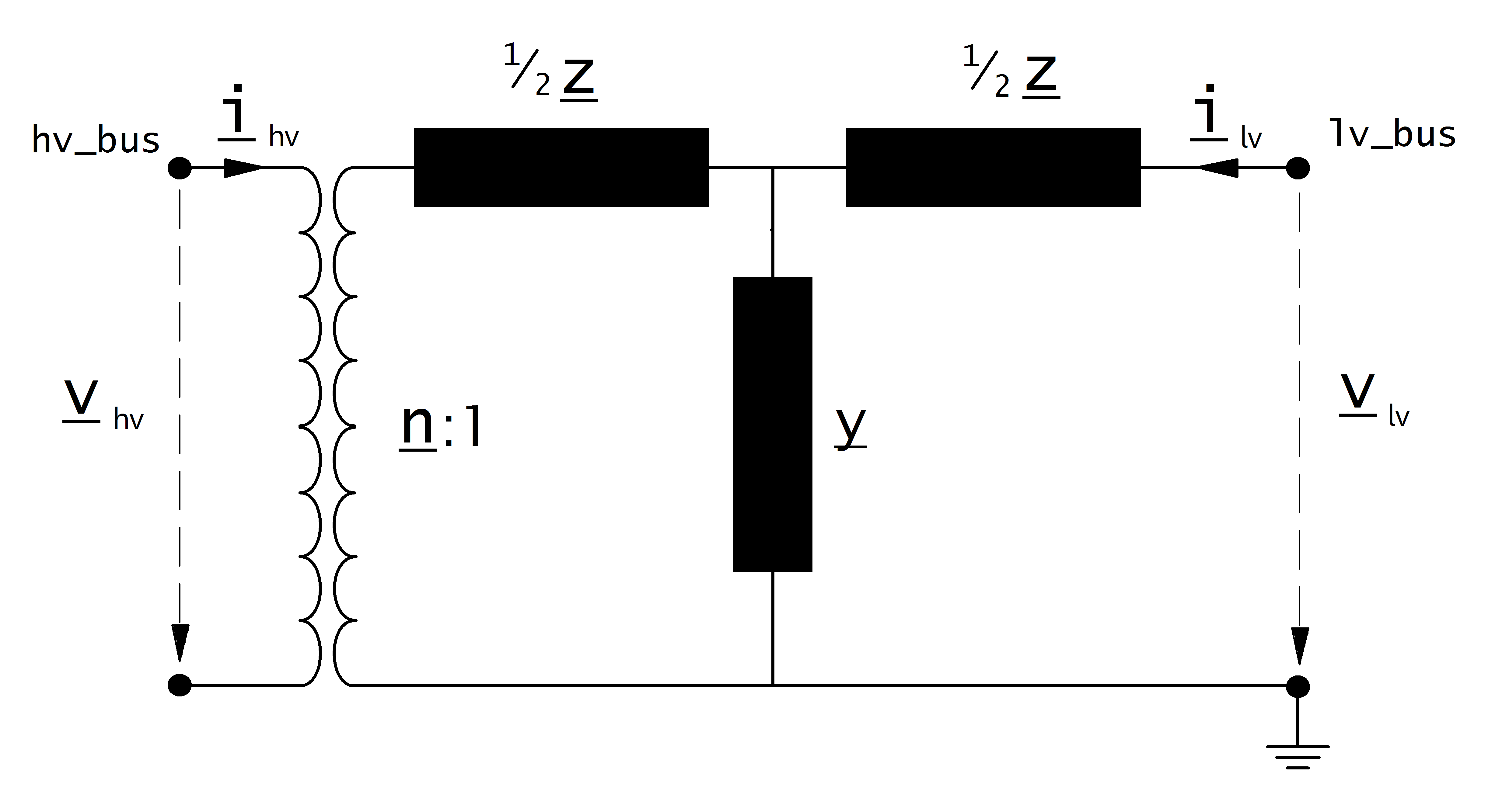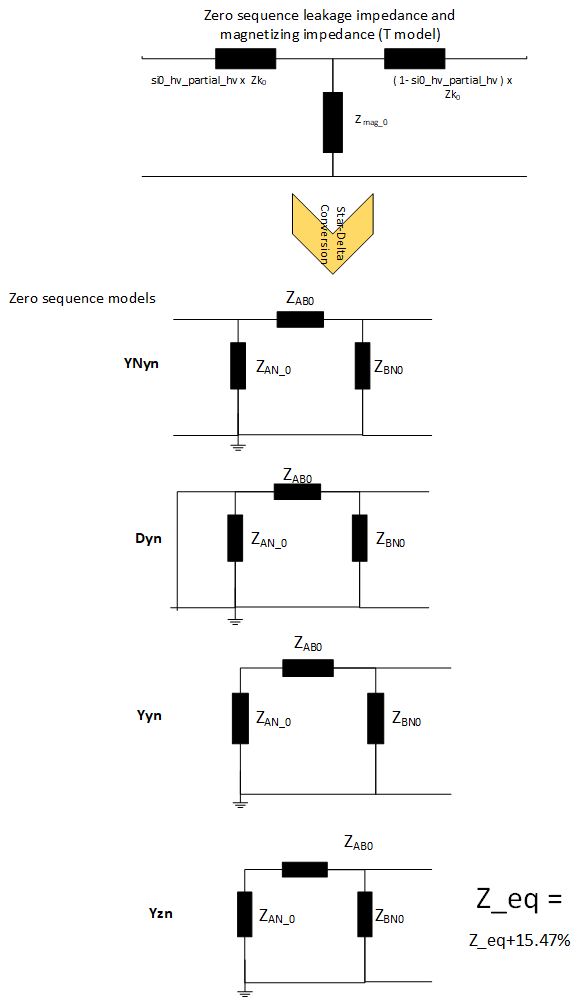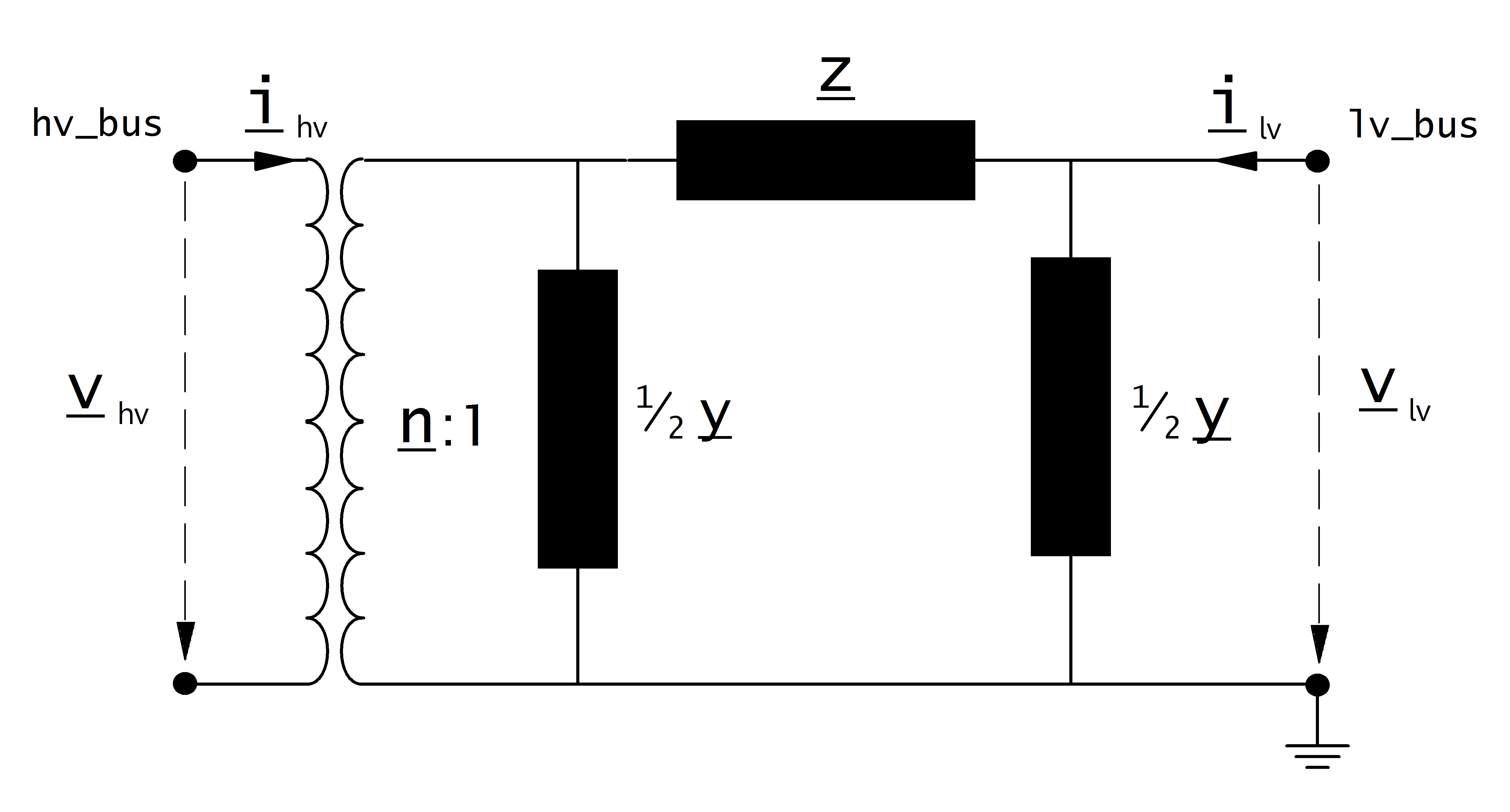# Transformer¶

## Create Function¶

Transformers can be either created from the standard type library (create_transformer) or with custom values (create_transformer_from_parameters).

pandapower.create_transformer(net, hv_bus, lv_bus, std_type, name=None, tap_pos=nan, in_service=True, index=None, max_loading_percent=nan, parallel=1, df=1.0, tap_dependent_impedance=None, vk_percent_characteristic=None, vkr_percent_characteristic=None, pt_percent=None, oltc=None, xn_ohm=None, **kwargs)

Creates a two-winding transformer in table net[“trafo”]. The trafo parameters are defined through the standard type library.

INPUT:

net - The net within this transformer should be created

hv_bus (int) - The bus on the high-voltage side on which the transformer will be connected to

lv_bus (int) - The bus on the low-voltage side on which the transformer will be connected to

std_type - The used standard type from the standard type library

Zero sequence parameters (Added through std_type For Three phase load flow) :

vk0_percent - zero sequence relative short-circuit voltage

vkr0_percent - real part of zero sequence relative short-circuit voltage

mag0_percent - ratio between magnetizing and short circuit impedance (zero sequence)

z_mag0 / z0

mag0_rx - zero sequence magnetizing r/x ratio

si0_hv_partial - zero sequence short circuit impedance distribution in hv side

OPTIONAL:

name (string, None) - A custom name for this transformer

tap_pos (int, nan) - current tap position of the transformer. Defaults to the medium position (tap_neutral)

in_service (boolean, True) - True for in_service or False for out of service

index (int, None) - Force a specified ID if it is available. If None, the index one higher than the highest already existing index is selected.

parallel (integer) - number of parallel transformers

df (float) - derating factor: maximal current of transformer in relation to nominal current of transformer (from 0 to 1)

tap_dependent_impedance (boolean) - True if transformer impedance must be adjusted dependent on the tap position of the trabnsformer. Requires the additional columns “vk_percent_characteristic” and “vkr_percent_characteristic” that reference the index of the characteristic from the table net.characteristic. A convenience function pandapower.control.create_trafo_characteristics can be used to create the SplineCharacteristic objects, add the relevant columns and set up the references to the characteristics. The function pandapower.control.trafo_characteristics_diagnostic can be used for sanity checks.

vk_percent_characteristic (int) - index of the characteristic from net.characteristic for the adjustment of the parameter “vk_percent” for the calculation of tap dependent impedance.

vkr_percent_characteristic (int) - index of the characteristic from net.characteristic for the adjustment of the parameter “vk_percent” for the calculation of tap dependent impedance.

xn_ohm (float) - impedance of the grounding reactor (Z_N) for shor tcircuit calculation

OUTPUT:

index (int) - The unique ID of the created transformer

EXAMPLE:

create_transformer(net, hv_bus = 0, lv_bus = 1, name = “trafo1”, std_type = “0.4 MVA 10/0.4 kV”)

pandapower.create_transformer_from_parameters(net, hv_bus, lv_bus, sn_mva, vn_hv_kv, vn_lv_kv, vkr_percent, vk_percent, pfe_kw, i0_percent, shift_degree=0, tap_side=None, tap_neutral=nan, tap_max=nan, tap_min=nan, tap_step_percent=nan, tap_step_degree=nan, tap_pos=nan, tap_phase_shifter=False, in_service=True, name=None, vector_group=None, index=None, max_loading_percent=nan, parallel=1, df=1.0, vk0_percent=nan, vkr0_percent=nan, mag0_percent=nan, mag0_rx=nan, si0_hv_partial=nan, pt_percent=None, oltc=None, tap_dependent_impedance=None, vk_percent_characteristic=None, vkr_percent_characteristic=None, xn_ohm=None, **kwargs)

Creates a two-winding transformer in table net[“trafo”]. The trafo parameters are defined through the standard type library.

INPUT:

net - The net within this transformer should be created

hv_bus (int) - The bus on the high-voltage side on which the transformer will be connected to

lv_bus (int) - The bus on the low-voltage side on which the transformer will be connected to

sn_mva (float) - rated apparent power

vn_hv_kv (float) - rated voltage on high voltage side

vn_lv_kv (float) - rated voltage on low voltage side

vkr_percent (float) - real part of relative short-circuit voltage

vk_percent (float) - relative short-circuit voltage

pfe_kw (float) - iron losses in kW

i0_percent (float) - open loop losses in percent of rated current

vector_group (String) - Vector group of the transformer

HV side is Uppercase letters and LV side is lower case

vk0_percent (float) - zero sequence relative short-circuit voltage

vkr0_percent - real part of zero sequence relative short-circuit voltage

mag0_percent - zero sequence magnetizing impedance/ vk0

mag0_rx - zero sequence magnitizing R/X ratio

si0_hv_partial - Distribution of zero sequence leakage impedances for HV side

OPTIONAL:

in_service (boolean) - True for in_service or False for out of service

parallel (integer) - number of parallel transformers

name (string) - A custom name for this transformer

shift_degree (float) - Angle shift over the transformer*

tap_side (string) - position of tap changer (“hv”, “lv”)

tap_pos (int, nan) - current tap position of the transformer. Defaults to the medium position (tap_neutral)

tap_neutral (int, nan) - tap position where the transformer ratio is equal to the ratio of the rated voltages

tap_max (int, nan) - maximal allowed tap position

tap_min (int, nan): minimal allowed tap position

tap_step_percent (float) - tap step size for voltage magnitude in percent

tap_step_degree (float) - tap step size for voltage angle in degree*

tap_phase_shifter (bool) - whether the transformer is an ideal phase shifter*

index (int, None) - Force a specified ID if it is available. If None, the index one higher than the highest already existing index is selected.

df (float) - derating factor: maximal current of transformer in relation to nominal current of transformer (from 0 to 1)

tap_dependent_impedance (boolean) - True if transformer impedance must be adjusted dependent on the tap position of the trabnsformer. Requires the additional columns “vk_percent_characteristic” and “vkr_percent_characteristic” that reference the index of the characteristic from the table net.characteristic. A convenience function pandapower.control.create_trafo_characteristics can be used to create the SplineCharacteristic objects, add the relevant columns and set up the references to the characteristics. The function pandapower.control.trafo_characteristics_diagnostic can be used for sanity checks.

vk_percent_characteristic (int) - index of the characteristic from net.characteristic for the adjustment of the parameter “vk_percent” for the calculation of tap dependent impedance.

vkr_percent_characteristic (int) - index of the characteristic from net.characteristic for the adjustment of the parameter “vk_percent” for the calculation of tap dependent impedance.

pt_percent (float, nan) - (short circuit only)

oltc (bool, False) - (short circuit only)

xn_ohm (float) - impedance of the grounding reactor (Z_N) for shor tcircuit calculation

** only considered in loadflow if calculate_voltage_angles = True

OUTPUT:

index (int) - The unique ID of the created transformer

EXAMPLE:

create_transformer_from_parameters(net, hv_bus=0, lv_bus=1, name=”trafo1”, sn_mva=40, vn_hv_kv=110, vn_lv_kv=10, vk_percent=10, vkr_percent=0.3, pfe_kw=30, i0_percent=0.1, shift_degree=30)

## Input Parameters¶

net.trafo

 Parameter Datatype Value Range Explanation name string name of the transformer std_type string transformer standard type name hv_bus* integer high voltage bus index of the transformer lv_bus* integer low voltage bus index of the transformer sn_mva* float $$>$$ 0 rated apparent power of the transformer [MVA] vn_hv_kv* float $$>$$ 0 rated voltage at high voltage bus [kV] vn_lv_kv* float $$>$$ 0 rated voltage at low voltage bus [kV] vk_percent* float $$>$$ 0 short circuit voltage [%] vkr_percent* float $$\geq$$ 0 real component of short circuit voltage [%] pfe_kw* float $$\geq$$ 0 iron losses [kW] i0_percent* float $$\geq$$ 0 open loop losses in [%] vk0_percent*** float $$\geq$$ 0 zero sequence relative short-circuit voltage vkr0_percent*** float $$\geq$$ 0 real part of zero sequence relative short-circuit voltage mag0_percent*** float $$\geq$$ 0 z_mag0 / z0 ratio between magnetizing and short circuit impedance (zero sequence) mag0_rx*** float zero sequence magnetizing r/x ratio si0_hv_partial*** float $$\geq$$ 0 zero sequence short circuit impedance distribution in hv side vector_group*** String ‘Dyn’,’Yyn’,’Yzn’,’YNyn’ Vector Groups ( required for zero sequence model of transformer ) shift_degree* float transformer phase shift angle tap_side string “hv”, “lv” defines if tap changer is at the high- or low voltage side tap_neutral integer rated tap position tap_min integer minimum tap position tap_max integer maximum tap position tap_step_percent float $$>$$ 0 tap step size for voltage magnitude [%] tap_step_degree float $$\geq$$ 0 tap step size for voltage angle tap_pos integer current position of tap changer tap_phase_shifter bool defines whether the transformer is an ideal phase shifter parallel int $$>$$ 0 number of parallel transformers max_loading_percent** float $$>$$ 0 Maximum loading of the transformer with respect to sn_mva and its corresponding current at 1.0 p.u. df float 1 $$\geq$$ df $$>$$ 0 derating factor: maximal current of transformer in relation to nominal current of transformer (from 0 to 1) in_service* boolean True / False specifies if the transformer is in service oltc* boolean True / False specifies if the transformer has an OLTC (short-circuit relevant) power_station_unit* boolean True / False specifies if the transformer is part of a power_station_unit (short-circuit relevant).

*necessary for executing a balanced power flow calculation
**optimal power flow parameter
***necessary for executing a three phase power flow / single phase short circuit

Note

Note

vkr_percent can be calculated as follow :

\begin{align*} vkr\_percent &= \frac{P\_cu}{S\_trafo} \cdot 100 \end{align*}
Where
P_cu is the power loss in the copper in kW
S_trafo is the rated apparent power of the transformer in kW

## Electric Model¶

The equivalent circuit used for the transformer can be set in the power flow with the parameter “trafo_model”.

trafo_model=’t’:sequence = 0:trafo_model=’pi’:### Transformer Ratio¶

The magnitude of the transformer ratio is given as:

\begin{align*} n &= \frac{V_{ref, HV, transformer}}{V_{ref, LV, transformer}} \cdot \frac{V_{ref, LV bus}}{V_{ref, HV bus}} \end{align*}

The reference voltages of the high- and low voltage buses are taken from the net.bus table. The reference voltage of the transformer is taken directly from the transformer table:

\begin{align*} V_{ref, HV, transformer} &= vn\_hv\_kv \\ V_{ref, LV, transformer} &= vn\_lv\_kv \end{align*}

If the power flow is run with voltage_angles=True, the complex ratio is given as:

\begin{align*} \underline{n} &= n \cdot e^{j \cdot \theta \cdot \frac{\pi}{180}} \\ \theta &= shift\_degree \end{align*}

Otherwise, the ratio does not include a phase shift:

\begin{align*} \underline{n} &= n \end{align*}

### Impedance Values¶

The short-circuit impedance is calculated as:

\begin{align*} z_k &= \frac{vk\_percent}{100} \cdot \frac{net.sn\_mva}{sn\_mva} \\ r_k &= \frac{vkr\_percent}{100} \cdot \frac{net.sn\_mva}{sn\_mva} \\ x_k &= \sqrt{z^2 - r^2} \\ \underline{z}_k &= r_k + j \cdot x_k \end{align*}

The magnetising admittance is calculated as:

\begin{align*} y_m &= \frac{i0\_percent}{100} \\ g_m &= \frac{pfe\_kw}{sn\_mva \cdot 1000} \cdot \frac{net.sn\_mva}{sn\_mva} \\ b_m &= \sqrt{y_m^2 - g_m^2} \\ \underline{y_m} &= g_m - j \cdot b_m \end{align*}

The values calculated in that way are relative to the rated values of the transformer. To transform them into the per unit system, they have to be converted to the rated values of the network:

\begin{align*} Z_{N} &= \frac{V_{N}^2}{S_{N}} \\ Z_{ref, trafo} &= \frac{vn\_lv\_kv^2 \cdot net.sn\_mva}{sn\_mva} \\ \underline{z} &= \underline{z}_k \cdot \frac{Z_{ref, trafo}}{Z_{N}} \\ \underline{y} &= \underline{y}_m \cdot \frac{Z_{N}}{Z_{ref, trafo}} \\ \end{align*}

Where the reference voltage $$V_{N}$$ is the nominal voltage at the low voltage side of the transformer and the rated apparent power $$S_{N}$$ is defined system wide in the net object (see Unit Systems and Conventions).

### Tap Changer¶

Longitudinal regulator

A longitudinal regulator can be modeled by setting tap_phase_shifter to False and defining the tap changer voltage step with tap_step_percent.

The reference voltage is then multiplied with the tap factor:

\begin{align*} n_{tap} = 1 + (tap\_pos - tap\_neutral) \cdot \frac{tap\_st\_percent}{100} \end{align*}

On which side the reference voltage is adapted depends on the $$tap\_side$$ variable:

 tap_side=”hv” tap_side=”lv” $$V_{n, HV, transformer}$$ $$vnh\_kv \cdot n_{tap}$$ $$vnh\_kv$$ $$V_{n, LV, transformer}$$ $$vnl\_kv$$ $$vnl\_kv \cdot n_{tap}$$

Note

The variables tap_min and tap_max are not considered in the power flow. The user is responsible to ensure that tap_min < tap_pos < tap_max!

Cross regulator

In addition to tap_step_percent a value for tap_step_degree can be defined to model an angle shift for each tap, resulting in a cross regulator that affects the magnitude as well as the angle of the transformer ratio.

Ideal phase shifter

If tap_phase_shifter is set to True, the tap changer is modeled as an ideal phase shifter, meaning that a constant angle shift is added with each tap step:

\begin{align*} \underline{n} &= n \cdot e^{j \cdot (\theta + \theta_{tp}) \cdot \frac{\pi}{180}} \\ \theta &= shift\_degree \end{align*}

The angle shift can be directly defined in tap_step_degree, in which case:

\begin{align*} \theta_{tp} = tap\_st\_degree \cdot (tap\_pos - tap\_neutral) \end{align*}

or it can be given as a constant voltage step in tap_step_percent, in which case the angle is calculated as:

\begin{align*} \theta_{tp} = 2 \cdot arcsin(\frac{1}{2} \cdot \frac{tap\_st\_percent}{100}) \cdot (tap\_pos - tap\_neutral) \end{align*}

If both values are given for an ideal phase shift transformer, the power flow will raise an error.

## Result Parameters¶

net.res_trafo

 Parameter Datatype Explanation p_hv_mw float active power flow at the high voltage transformer bus [MW] q_hv_mvar float reactive power flow at the high voltage transformer bus [MVar] p_lv_mw float active power flow at the low voltage transformer bus [MW] q_lv_mvar float reactive power flow at the low voltage transformer bus [MVar] pl_mw float active power losses of the transformer [MW] ql_mvar float reactive power consumption of the transformer [Mvar] i_hv_ka float current at the high voltage side of the transformer [kA] i_lv_ka float current at the low voltage side of the transformer [kA] vm_hv_pu float voltage magnitude at the high voltage bus [pu] vm_lv_pu float voltage magnitude at the low voltage bus [pu] va_hv_degree float voltage angle at the high voltage bus [degrees] va_lv_degree float voltage angle at the low voltage bus [degrees] loading_percent float load utilization relative to rated power [%]
\begin{align*} p\_hv\_mw &= Re(\underline{v}_{hv} \cdot \underline{i}^*_{hv}) \\ q\_hv\_mvar &= Im(\underline{v}_{hv} \cdot \underline{i}^*_{hv}) \\ p\_lv\_mw &= Re(\underline{v}_{lv} \cdot \underline{i}^*_{lv}) \\ q\_lv\_mvar &= Im(\underline{v}_{lv} \cdot \underline{i}^*_{lv}) \\ pl\_mw &= p\_hv\_mw + p\_lv\_mw \\ ql\_mvar &= q\_hv\_mvar + q\_lv\_mvar \\ i\_hv\_ka &= i_{hv} \\ i\_lv\_ka &= i_{lv} \end{align*}

net.res_trafo_3ph

 Parameter Datatype Explanation p_a_hv_mw float active power flow at the high voltage transformer bus : Phase A [MW] q_a_hv_mvar float reactive power flow at the high voltage transformer bus : Phase A [MVar] p_b_hv_mw float active power flow at the high voltage transformer bus : Phase B [MW] q_b_hv_mvar float reactive power flow at the high voltage transformer bus : Phase B [MVar] p_c_hv_mw float active power flow at the high voltage transformer bus : Phase C [MW] q_c_hv_mvar float reactive power flow at the high voltage transformer bus : Phase C [MVar] p_a_lv_mw float active power flow at the low voltage transformer bus : Phase A [MW] q_a_lv_mvar float reactive power flow at the low voltage transformer bus : Phase A [MVar] p_b_lv_mw float active power flow at the low voltage transformer bus : Phase B [MW] q_b_lv_mvar float reactive power flow at the low voltage transformer bus : Phase B [MVar] p_c_lv_mw float active power flow at the low voltage transformer bus : Phase C [MW] q_c_lv_mvar float reactive power flow at the low voltage transformer bus : Phase C [MVar] pl_a_mw float active power losses of the transformer : Phase A [MW] ql_a_mvar float reactive power consumption of the transformer : Phase A [Mvar] pl_b_mw float active power losses of the transformer : Phase B [MW] ql_b_mvar float reactive power consumption of the transformer : Phase B [Mvar] pl_c_mw float active power losses of the transformer : Phase C [MW] ql_c_mvar float reactive power consumption of the transformer : Phase C [Mvar] i_a_hv_ka float current at the high voltage side of the transformer : Phase A [kA] i_a_lv_ka float current at the low voltage side of the transformer : Phase A [kA] i_b_hv_ka float current at the high voltage side of the transformer : Phase B [kA] i_b_lv_ka float current at the low voltage side of the transformer : Phase B [kA] i_c_hv_ka float current at the high voltage side of the transformer : Phase C [kA] i_c_lv_ka float current at the low voltage side of the transformer : Phase C [kA] loading_percent float load utilization relative to rated power [%]
\begin{align*} p\_hv\_mw_{phase} &= Re(\underline{v}_{hv_{phase}} \cdot \underline{i}^*_{hv_{phase}}) \\ q\_hv\_mvar_{phase} &= Im(\underline{v}_{hv_{phase}} \cdot \underline{i}^*_{hv_{phase}}) \\ p\_lv\_mw_{phase} &= Re(\underline{v}_{lv_{phase}} \cdot \underline{i}^*_{lv_{phase}}) \\ q\_lv\_mvar_{phase} &= Im(\underline{v}_{lv_{phase}} \cdot \underline{i}^*_{lv_{phase}}) \\ pl\_mw_{phase} &= p\_hv\_mw_{phase} + p\_lv\_mw_{phase} \\ ql\_mvar_{phase} &= q\_hv\_mvar_{phase} + q\_lv\_mvar_{phase} \\ i\_hv\_ka_{phase} &= i_{hv_{phase}} \\ i\_lv\_ka_{phase}&= i_{lv_{phase}} \end{align*}